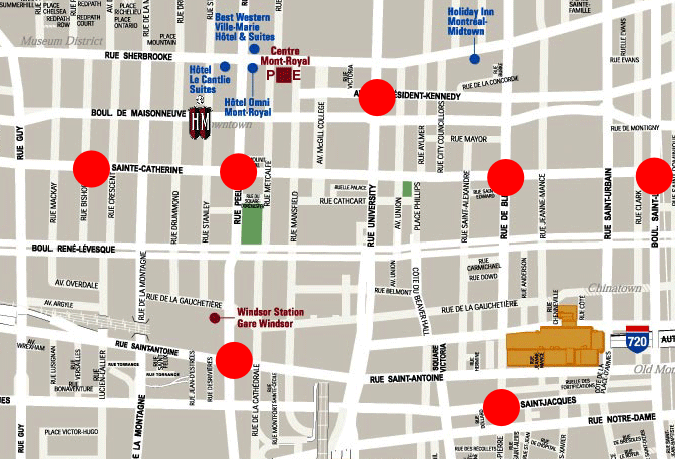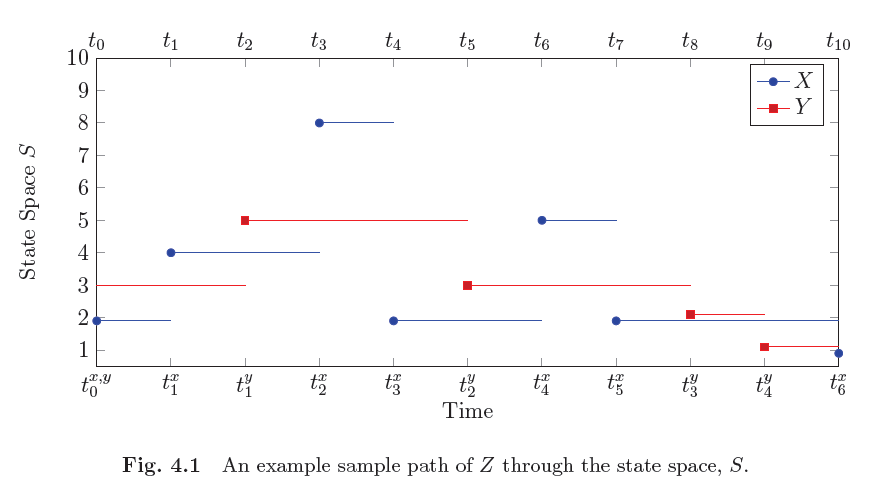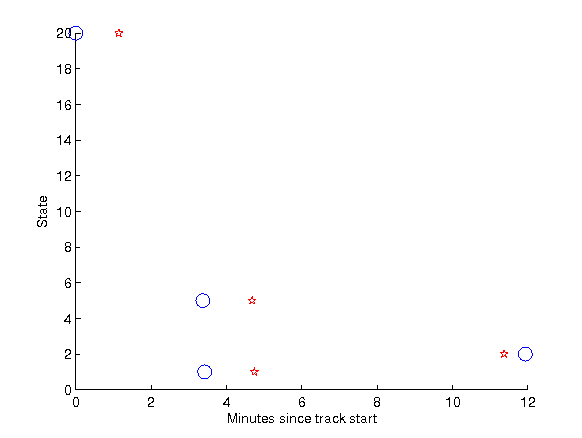## Convoy Detection in Networks of Short-Range SensorsDetecting groups of vechicles travelling together as a convoy is an important problem in military and law enforcement applications. We present a method for identifying these groups travelling together over time using short-range sensors distributed over a road network. Results of expirements on simulated and real data show that our algorithm does detect and report convoys in real-time. The figure shows an example convoy found in a real dataset.

Ph.D. Students: Sean Lawlor

Faculty: Prof. Michael RabbatThe system presented uses license plate recognition (LPR) cameras distributed across a city in order to receive information about where a vehicle was recognized and the time of recognition. Using this data we construct a semi-Markov model describing how vehicles move between the state (sensor) locations. We then construct two models for vehicles travelling independently as well as in a convoy where transitions in the semi-Markov process are correlated. From these two models, a sequential hypothesis test is constructed which updates the likelihood ratio of the process travelling as a convoy as samples of each vehicle are received. The image to the right shows example sensor locations overlayed on a city grid.This model represents the joint random process, Z, of two random objects X and Y travelling through the state space (set of sensors). Each state has a corresponding latitude and longitude location. We have no knowledge of where the vehicles are in-between samples at sensor locations, leading to the Markov model where it is assumed they do not move until observed next.

Each joint process is a combination of two individual processes travelling through the Markov chain. Each process are characterized by observations at each individual state and the time of the transition to that state, more specifically
$$\mathbf{X} = \left\lbrace X(t^x_0) = x_o, X(t^x_1) = x_1,...,X(t^x_{n_x(t)}) = x_{n_x(t)} \right\rbrace$$
where $n_x(t)$ is the number of observations of $X$ at time $t$, or
$$n_x(t) = \max \lbrace k : t_{k}^{x} \leq t \rbrace .$$

The model then defines the joint process as
$$Z(t) = \left[ X(t^x_{n_x(t)}), Y(t^y_{n_y(t)}) \right]$$
which captures the current state of objects $X$ and $Y$ at the time $t$.

We now provide a method of defining the probability that two objects, $X$ and $Y$, are travelling independently in model $H_0$ (which simply the Markov probability for each object). We also define a density describing the two objects travelling dependently, in model $H_1$, taking into account the physical properties of the transitions being made. We then define a sequential hypothesis test as
$$\Lambda \left( Z(t_i) = z_i | Z(t_{i-1}) = z_{i-1} \right) = \frac{\Pr \left( Z(t_i) = z_i | Z(t_{i-1}) = z_{i-1}, H_1 \right)}{\Pr \left( Z(t_i) = z_i | Z(t_{i-1}) = z_{i-1}, H_0 \right)} .$$
The decision rules for this likelihood ratio are the following

 $\Lambda \left( Z(t_i) = z_i | Z(t_{i-1}) = z_{i-1} \right)$ < $\eta_0$ decide $H_0$ $\eta_0$ $\leq$ $\Lambda \left( Z(t_i) = z_i | Z(t_{i-1}) = z_{i-1} \right)$ < $\eta_1$ decide "need more data" $\eta_1$ $\leq$ $\Lambda \left( Z(t_i) = z_i | Z(t_{i-1}) = z_{i-1} \right)$ decide $H_1$

where the quantities $\eta_0$ and $\eta_1$ are chosen to control the probability of false detection ($P_{FD}$) and probability of detection ($P_{D}$).

An example detected convoy can be seen in the followingThe example path of a detected convoy through space. The z-axis denotes the minutes since the track of the pair started. The x and y-axes denote latitude and longitude. This is the same convoy as in the path plot however instead of plotting the actualy latitude and longitue we have plotted the states where the object was observed.

Lawlor, S. and M. G. Rabbat, "Detecting Convoys in Networks of Short-Range Sensors", 2014 Asilomar Conference on Signals, Systems, and Computers, Pacific Grove, California, 11/2014

Lawlor, S, "Detecting Convoys in Networks of Short-Range Sensors", Electrical and Computer Engineering, Masters of Engineering, Montreal, Quebec, McGill University, pp. 73, 08/2013.